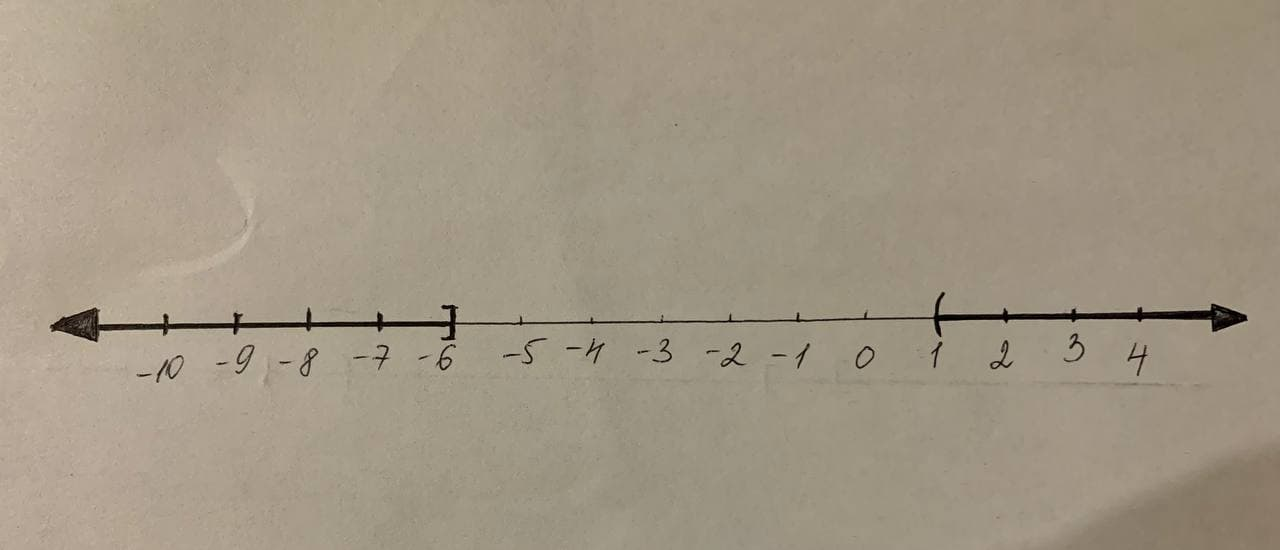# To calculate: The solution of the compound inequality -2\left(x-1\right)+4<x+3\ \textmronjo7n 2021-11-22 Answered

To calculate: The solution of the compound inequality
$$\displaystyle-{2}{\left({x}-{1}\right)}+{4}{<}{x}+{3}\ r \ 5\left(x+2\right)-3\le4x+{r}\ {5}{\left({x}+{2}\right)}-{3}\le{4}{x}+{1}$$
and graph the solution set and write the solution set in interval notation.

• Questions are typically answered in as fast as 30 minutes

### Plainmath recommends

• Get a detailed answer even on the hardest topics.
• Ask an expert for a step-by-step guidance to learn to do it yourself.jackbranv5

Given information:
The compound inequality, $$\displaystyle-{2}{\left({x}-{1}\right)}+{4}{<}{x}+{3}\ r \ 5\left(x+2\right)-3\le4x+{r}\ {5}{\left({x}+{2}\right)}-{3}\le{4}{x}+{1}$$.
Formula used:
Steps to solve compound inequality:
Step 1: First, solve the compound inequality individually.
Step 2: If two inequalities are combined through “or’/“and” then take the union/intersection, respectively, of individual solution set.
Calculation:
The given compound inequality is $$\displaystyle-{2}{\left({x}-{1}\right)}+{4}{<}{x}+{3}\ r \ 5\left(x+2\right)-3\le4x+{r}\ {5}{\left({x}+{2}\right)}-{3}\le{4}{x}+{1}$$. Simplify the inequality.
$$\displaystyle-{2}{\left({x}-{1}\right)}+{4}{<}{x}+{3}\ r \ 5\left(x+2\right)-3\le4x+{r}\ {5}{\left({x}+{2}\right)}-{3}\le{4}{x}+{1}$$
$$\displaystyle-{2}{x}+{2}+{4}{<}{x}+{3}\ r \ 5x+10-3\le4x+{r}\ {5}{x}+{10}-{3}\le{4}{x}+{1}$$
$$\displaystyle-{2}{x}-{x}{<}{3}-{6}\ r \ 5x-4x\le1-{r}\ {5}{x}-{4}{x}\le{1}-{7}$$
$$\displaystyle{x}{>}{1}\ r \ x\le-{r}\ {x}\le-{6}$$
The graph of the solution is:
Therefore, the solution set of compound inequality
$$\displaystyle-{2}{\left({x}-{1}\right)}+{4}{<}{x}+{3}\ r \ 5\left(x+2\right)-3\le4x+{r}\ {5}{\left({x}+{2}\right)}-{3}\le{4}{x}+{1}$$ in interval notation is:
$$(-\infty-6) \cup(1,\infty)$$.

###### Have a similar question?Thomas Conway

Given information:
The compound inequality, $$-2\left(x-1\right)+4<x+3\ \text or \ 5\left(x+2\right)-3\le4x+1$$.
Formula used:
Steps to solve compound inequality:
Step 1: First, solve the compound inequality individually.
Step 2: If two inequalities are combined through “or’/“and” then take the union/intersection, respectively, of individual solution set.
Calculation:
The given compound inequality is $$-2\left(x-1\right)+4<x+3\ \text or \ 5\left(x+2\right)-3\le4x+1$$. Simplify the inequality.
$$-2\left(x-1\right)+4<x+3\ \text or \ 5\left(x+2\right)-3\le4x+1$$
$$-2x+2+4<x+3 \ \text or \ 5x+10-3\le4x+1$$
$$-2x-x<3-6 \ \text or \ 5x-4x\le1-7$$
$$x>1 \ \text or \ x\le-6$$
The graph of the solution is:Therefore, the solution set of compound inequality $$-2\left(x-1\right)+4<x+3\ \text or \ 5\left(x+2\right)-3\le4x+1$$ in interval notation is: $$\left(-\propto-6\mid\cup\left(1,\propto\right)\right)$$.
Given information: